# Rectangle + ratio - math problems

#### Number of problems found: 60

• A rectangle 8A rectangle measuring 6 cm and 4 cm is enlarged by the ratio 3:1. What is the area of the enlarged rectangle?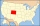The state Colorado has the shape of a rectangle. The map is sold on 2 posters. The first has dimensions of 70 cm and 50 cm in a scale of 1: 1,000,000. The second poster has dimensions of 175 cm and 125 cm. What is the scale of the second poster?
• Sides of a rectangleThe dimensions of a rectangle are in a 4:12 ratio. If the shorter side length is 12 cm, what is the length of the longer side in centimetres?
• A rectangle 4A rectangle has area 300 and perimeter 80. what is the ratio of the length and width?
• An architectAn architect makes a model of a new house. The model shows a tile patio in the backyard. In the model, each tile has a length of 1/2 inch and a width of 1/6 inch. The actual tiles have a length of 2/3 feet and a width of 2/9 feet. What is the ratio of the
• The circumferenceThe circumference and width of the rectangle are in a ratio of 5: 1. its area is 216cm2. What is its length?
• Cuboid wallsThe block's base is a rectangle whose sides have lengths in the ratio of 13: 7. Find the volume of the block in liters if the longer side of the base measures 65 cm, and the height of the block is 1.2 m
• Negative and postcard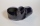The image on the negative has dimensions of 36x24 mm and is enlarged to a postcard format of 13.5x9 cm. In what proportion have the lengths increased? In what proportion has the image content increased?
• Two bodiesThe rectangle with dimensions 8 cm and 4 cm is rotated 360º first around the longer side to form the first body. Then, we similarly rotate the rectangle around the shorter side b to form a second body. Determine the ratio of surfaces of the first and seco
• A rectangle 2A rectangle has a diagonal length of 74cm. Its side lengths are in ratio 5:3. Find its side lengths.
• Plan scale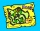The actual width of the room is 66 dm, the width in plan is 4.4 cm. What scale corresponds to the plan?
• Two rectangles 2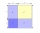A square of area 36 cm2 is cut out to make two rectangles. A and B The area of area A to area B is 2 : 1 Find the dimensions of rectangles A and B.
• The perimeter 3The perimeter of a rectangle is 35 cm. The ratio of the length to its width is 3:2. Calculate the dimensions of the rectangle
• Ratio of sidesCalculate the area of a circle with the same circumference as the circumference of the rectangle inscribed with a circle with a radius of r 9 cm so that its sides are in ratio 2 to 7.
• Three gardensIs it possible to divide a plot of land with an area of 920 m2 into three gardens in a ratio of 4: 2: 3 so that their areas are only whole multiples of square meters?
• Area of rectangleHow many times will increase the area of the rectangle if we increase twice the length and at the same time we decrease the width by half?
• Rectangular plotThe dimensions of a rectangular plot are (x+1)m and (2x-y)m. If the sum of x and y is 3m and the perimeter of the plot is 36m. Find the area of the diagonal of the plot.
• Rectangular fieldA rectangular field has a diagonal of length 169m. If the length and width are in the ratio 12:5. Find the dimensions of the field, the perimeter of the field and the area of the field.
• Rectangular gardenThe sides of the rectangular garden are in ratio 1: 2. The diagonal has a length of 20 meters. Calculate the area and perimeter of the garden.
• Square to rectangle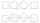What is the ratio of the area of a square of side x to the area of a rectangle of a rectangle of width 2 x and length 3

Do you have an exciting math question or word problem that you can't solve? Ask a question or post a math problem, and we can try to solve it.

We will send a solution to your e-mail address. Solved examples are also published here. Please enter the e-mail correctly and check whether you don't have a full mailbox.

Check out our ratio calculator. Rectangle Problems. Ratio - math problems.